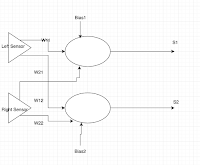## Friday 23 December 2016

### 4Tronix Bit:Bot Neuron Controlled Edge follower

In the last post I was playing with 4Tronix's Bit:Bot. In this post I will show the initial experimentation with an artificial neuron controlling the Bit:Bot to follow the edge of a line (it follows the left-hand side of the line).The neurons (well two separate ones, S1 and S2) are produced using weighted sums - summing the weights x inputs [ right-hand sensor (rs) and left-hand sensor (ls)] plus a bias for each neuron in this case w and w.

net=w+w*rs+w*ls
net2=w+w*rs+w*ls

If weighted sum >=0 then its output 1 otherwise 0
if net>=0:
s1=1
else:
s1=0

if net2>=0:
s2=1
else:
s2=0

What actual causes S1 to be either 1 or 0 is all defined by a set of weights w (three for the first neurone, S1,  three for S2).

w=[0,-1,1,-1,1,-1]

Converting the outputs of the two neurones S1 and S2 into actions is shown below.
if s1==1 and s2==1:
forward(40)
elif s1==0 and s2==1:
forward(30)
right_turn(10)
elif s1==1 and s2==0:
forward(30)
left_turn(10)
elif s1==0 and s2==0:
backward(40)

The functions for forward, right_turn, etc are defined elsewhere.

At the moment the movement is a bit rough and it is a little simpler to build a version that follows the centre of the line; this approach though, works with thinner lines.

To change the function of the system, change the values in w; for example to produce one that follows the centre of the line just change w (I will leave that to someone to work on). The complete code is shown below.

Code
from microbit import *
import neopixel, random, array

w=[]

def forward(n):
pin0.write_analog(551)
pin8.write_digital(0)
pin1.write_analog(551)
pin12.write_digital(0)
sleep(n)

def backward(n):
pin0.write_analog(551)
pin8.write_digital(1)
pin1.write_analog(551)
pin12.write_digital(1)
sleep(n)

def right_turn(n):
pin0.write_analog(511)
pin8.write_digital(0)
pin1.write_analog(511)
pin12.write_digital(1)
sleep(n)

def left_turn(n):
pin0.write_analog(551)
pin8.write_digital(1)
pin1.write_analog(551)
pin12.write_digital(0)
sleep(n)

w=[0,-1,1,-1,1,-1]

while True:

net=w+w*rs+w*ls
net2=w+w*rs+w*ls

if net>=0:
s1=1
else:
s1=0

if net2>=0:
s2=1
else:
s2=0

if s1==1 and s2==1:
forward(40)
elif s1==0 and s2==1:
forward(30)
right_turn(10)
elif s1==1 and s2==0:
forward(30)
left_turn(10)
elif s1==0 and s2==0:
backward(40)

All opinions in this blog are the Author's and should not in any way be seen as reflecting the views of any organisation the Author has any association with. Twitter @scottturneruon

1.This comment has been removed by a blog administrator.

1.